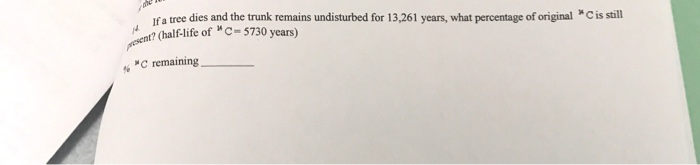# Question & Answer: If a tree dies and the trunk remains undisturbed for 13,261 years, what percentage of original…..Please answer and explain! Thank you!

If a tree dies and the trunk remains undisturbed for 13,261 years, what percentage of original ^14C is still present? (half-life of ^14C = 5730 years) % ^14C remaining _____

time = 13261 years

Don't use plagiarized sources. Get Your Custom Essay on
Question & Answer: If a tree dies and the trunk remains undisturbed for 13,261 years, what percentage of original…..
GET AN ESSAY WRITTEN FOR YOU FROM AS LOW AS \$13/PAGE

find % of C14 still present

so

the half life equation for decay:

Cfinal = Cinitial * (1/2) * (time / Half life)

the half life = 5730 years, the time = 13261 years

assume Cfinal /Cinitial is the fraction

so

Cfinal/Cinitial = * (1/2) ^ (time / Half life)

Cfinal/Cinitial = (1/2) ^ (13261/5730)

Cfinal/Cinitial =0.20105

then, only 0.201 fraciton is lefft, which is equivalent to 20.1 %

remainig C14% = 20.1 %